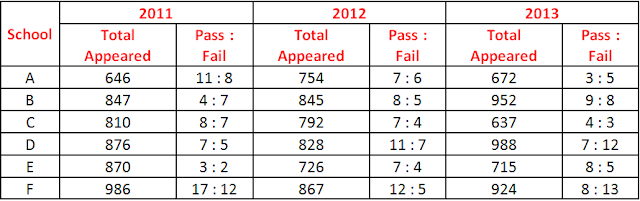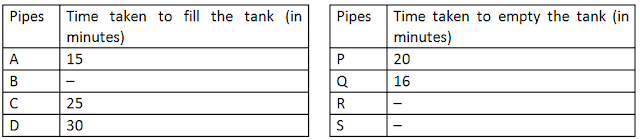# Quantitative Aptitude Practice Questions for IBPS Clerk – Set 10

Quantitative Aptitude Practice Questions for IBPS Clerk – Set 10
Directions (1 – 5): Study the following information carefully and answer the questions given beside:
The following table shows the total number of students appeared in an entrance exam from different schools in three different years, and the ratio of passed to failed students among them.  Answer the given questions based on the table given below.1. What is the difference between the number of passed students from School D in the year 2011 and the total number of failed students from School B in the year 2013?
a) 56
b) 60
c) 63
d) 68
e) 72
2. What is the total number of failed students from School F in all three years together?
a) 1145
b) 1235
c) 1325
d) 1415
e) 1525
3. What is the average number of failed students from School C in all three years together?
a) 311
b) 312
c) 313
d) 314
e) 315
4. The total number of passed students from school E in the year 2011 is approximately what per cent of the total number of failed students from School A in the year 2012?
a) 166.16%
b) 80%
c) 112.5%
d) 137%
e) None of these
5. What is the total number of passed students from all six schools in the year 2012?
a) 2850
b) 2940
c) 2930
d) 3010
e) 3510
Directions (6 – 10): Study the following pie-charts carefully and answer the questions given beside:
Pie-chart I shows discipline-wise breakup of the number of students who appeared in the university entrance examination and pie-chart II shows discipline wise breakup and the number of students selected by the university.
6. What is the difference between the number of students selected from Engineering discipline and the number of students selected from Others discipline?
a) 207
b) 184
c) 315
d) 295
e) 267
7. For which discipline is the difference between the number of students selected and the number of students appearing in the examination the minimum?
a) Agriculture
b) management
c) Science
d) Others
e) Commerce
8. The total number of candidates appearing from Science and Others disciplines was what per cent of the number of candidates appearing from Engineering and Commerce disciplines?
a) 117%
b) 108%
c) 140.63%
d) 129.33%
e) None of these
9. What was the ratio of the number of students appearing in examination from Management discipline to the number of students selected from Engineering discipline?
a) 19 : 17
b) 187 : 184
c) 23 : 21
d) 105 : 97
e) None of these
10. The number of students selected in Commerce and Management disciplines together is what per cent of the number of students appearing in Science and Agriculture disciplines together?
a) 24.39%
b) 28.32%
c) 27.93%
d) 31.74%
e) 36.21%
Directions (11 – 15): Study the following table to answer the questions that follow. Some values are missing. Study the table carefully and answer the questions based on data in table and particular questions.11. To fill a tank, pipes A and C were opened at 2:10 PM. After 5 minutes pipe A was closed and at the same time pipe P got opened. After some time it was found that tank was 2/3rd empty, at what time it was observed?
a) 2:32 PM
b) 2:30 PM
c) 2:27 PM
d) 2:35 PM
e) 2:20 PM
12. A tank was 2/5th full. To fill it more, pipe D was to be opened, but accidentally pipe Q got opened. When it was noticed after 4 minutes, pipe Q was closed. And now to fill tank quickly, pipe C was opened. What time could have been saved if pipe Q was not opened?
a) 7.45 minutes
b) 5.35 minutes
c) 5.25 minutes
d) 8.35 minutes
e) 7.25 minutes
13. There was some garbage in a tank. To remove the garbage, pipes A and R worked alternately for 3 minutes each starting with A. The procedure continued for 24 minutes, after which pipes A and D were opened to fill the tank. If the tank was filled in next 7 minutes, find the time in which pipe R can empty the full tank.
a) 24 minutes
b) 28 minutes
c) 18 minutes
d) 20 minutes
e) 32 minutes
14. A tank is to be filled by using pipes B and D simultaneously. After 9 minutes, some garbage was observed in tank so pipes B and D were closed and pipe S was opened. After 6 minutes, it was found, that tank is clean so pipe S was closed and again pipes B and D were opened, after which they filled the tank in 6 minutes. If pipe S alone can empty 2/9th tank in 4 minutes, in how much time pipe B can fill the fill tank?
a) 20 minutes
b) 16 minutes
c) 15 minutes
d) 24 minutes
e) 18 minutes
15. Using the data from table and above questions, which of the following combination of pipes will fill the tank in less time as compared to other combinations given?
a) A-B-S
b) B-C-P
c) A-D-Q
d) A-B-P
e) B-D-R

Solutions:
1. C) Passed students from School D in the year 2011 = 876 × 7/12 = 511
Failed students from school B in the year 2013 = 952 × 8/17
Reqd. difference = 511 – 448 = 63.
2. B) Total no. of failed students from School F = 986 × 12/29 + 867 × 5/17 + 924 × 13/21 = 408 + 255 + 572 = 1235
3. C) Total no. of failed students from School C = 810 × 7/15 + 792 × 4/11 + 637 × 3/7 = 378 + 288 + 273 = 939
Reqd. avg = 939/3 = 313
4. E)  Reqd % = [870 × 3/5]/[754 × 6/13] × 100 = 522/348 × 100 = 150%
5. D) Total number of passed students from all six schools in the year 2012
= 754 × 7/13 + 845 × 8/13 + 792 × 7/11 + 828 × 11/18 + 726 × 7/11 + 867 × 12/17
= 406 + 520 + 504 + 506 + 462 + 612 = 3010
6. B) Reqd. difference = Percent of students in (Engineering discipline – Others discipline) of total students
=  (20% – 18%) of 9200 =  2% of 9200 = 9200 × 2/100 = 184
7. A) Difference in
Science = 18700 × 18/100 – 9200 × 23/100 = 1250
Commerce = 18700 × 15/100 – 9200 × 20/100 = 1701
Engineering = 18700 × 17/100 – 9200 × 20/100 = 1339
Management = 18700 × 10/100 – 9200 × 8/100 = 1134
Agriculture = 18700 × 13/100 – 9200 × 19/100 = 683
Others = 18700 × 27/100 – 9200 × 18/100 = 3393
Hence, It is clear that the difference between the appearing students and selected students is minimum in Agriculture
8. C) Reqd. % = (18 + 27)/(17 + 15) × 100 = 140.62%
9. B) Reqd ratio = (18700 × 10)/100 : (9200 × 20)/100 = 1870 : 1840 = 187 : 184
10. D) Reqd. % = [(9200 × 20)/100]/[(18700 × 31)/100] × 100 = 31.74%
11. D) A and C work for 5 minutes – (1/15 + 1/25) * 5 = 8/15 tank fill
Now A was closed and C and P work
In 1 minute they work = (1/25 – 1/20) = -1/100 — means empty
let they C and P work for x minutes up to when tank was found 2/3rd empty
So
8/15 + (-1/100)*x = 1/3        ….   [ 1/3rd tank is fill at this time]
Solve,  x = 2o mins
So the time when 2/3rd empty tank was observed – 2:10 + 5 minutes + 20 minutes = 2:35 PM
12. E) Tank was 2/5th full. So to fill 3/5th tank, D will take 3/5 * 30 = 18 minutes …………………….(1)
Now
Pipe Q was opened for 4 minutes, so it emptied = 1/16 * 4 = 1/4th tank
Now tank filled is = 2/5 – 1/4 = 3/20
Now pipe C was opened, to fill 17/20th tank, C takes = 17/20 * 25 = 85/4  = 21.25 minutes
So total time now taken to fill tank = 4 + 21.25 = 25.25 minutes  ……………………..(2)
So time that could have been saved = 25.25 – 18 = 7.25 minutes
13. A) Procedure continued for 24 minutes, so pipes A and R both worked for 12 minutes each, giving 3 minutes each in turn.
So in 12 minutes, pipe A’s work = 1/15 *12 = 4/5
In 12 minutes, pipe R’s work = 1/x *12 = 12/x       [Let x = time in which pipe R can empty the tank]
Now A and D worked for 7 minutes, so their work in 7 minutes = (1/15 + 1/30) * 7 = 7/10
So
4/5 – 12/x + 7/10 = 1
Solve, x = 24 minutes
14. E) S can empty 2/9th tank in 4 minutes, so full tank in 9/2 * 4 = 18 minutes
So now
(1/B + 1/30) * 9 + (-1/18) *6 + (1/B + 1/30) * 6 = 1
(1/B + 1/30) * 15 = 1 + 1/3
(1/B + 1/30) * 15 = 4/3
Solve, B = 18
15. D) A) A-B-S
[1/15 + 1/18 – 1/18] — 1/15 so this combination will fill tank in 15 minutes
B) B-C-P
[1/18 + 1/25 – 1/20] — 41/900 so this combination will fill tank in 900/41 = 21.9 minutes
Similarly
C) A-D-Q — 80/3  = 26.6 minutes
D) A-B-P — 180/13  = 13.8 minutes
E) B-D-R — 360/17 = 21.2 minutes